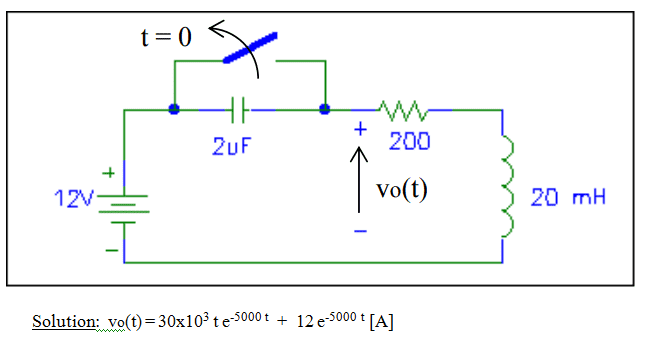# RLC circuit solved with Laplace transformation

• Engineering
• MaxR2018
In summary, the conversation is about solving an exercise involving Laplace's transformation and calculating V0(t) for t ≥ 0. The speaker made a mistake with the signs but was able to find the correct solutions for I(s) and v(s). The confusion was cleared up when they realized that the exercise was asking for the voltage in R and L together, not just VL.

#### MaxR2018

New poster has been reminded to use the Homework Help Template in the schoolwork forums
Hi, i need some help here. Can you help me?Here is the problem.

Exercise statement: The switch have been closed for a long time y is opened at t=0. Using Laplace's transtormation calculate V0(t) for t ≥ 0This is what i made to solve it:

1) I know while the switch is closed, the current trough the circuit is i=12v/200, so i=60mA.

2) When the switch is opened at t=0, i use the voltages law of kirchoff:

12 = vc + vr + vl

12= (1/c)*(integrate of i dt from 0 to t) + vc(0) + iR + L(di/dt)

i know that vc(0) = 0

so : 12= (1/c)*(integrate of i dt from 0 to t) + iR + L(di/dt)

Then i used the laplace transformation:

12/s = I(s)/Sc + RI(S) + LSI(S) - LI(0)

And i know that LI(0)=60mA

so:

12/s = I(s)/Sc + RI(S) + LSI(S) - 60mA

Finally i calculate I(S) and then i obtain i(t) with the antitransformation of Laplace.

Then, with i(t) i calculate vt knowing that:

VL=L*(di/dt), but i obtain a diferent solution.

I obtain that V0(t) = -300000*e^(-5000t) + 12e^(-5000t)

What I am doing wrong??

I thing I am having a mistake with some signs.

Pd: Sorry for my bad english.

#### Attachments

What do you get for the Laplace Transform of the current I(s)?

Last edited:
For I(s), I get:

$$I(s)=\frac{300}{(s+5000)^2}+\frac{0.06}{(s+5000)}$$
and, for v(s), I get $$v(s)=\frac{30000}{(s+5000)^2}+\frac{12}{(s+5000)}$$

•scottdave
Chestermiller said:
For I(s), I get:

$$I(s)=\frac{300}{(s+5000)^2}+\frac{0.06}{(s+5000)}$$
and, for v(s), I get $$v(s)=\frac{30000}{(s+5000)^2}+\frac{12}{(s+5000)}$$

One time you have I(s), you find V(s) with V(s) = LSI(S) - Li(0) ??

MaxR2018 said:
One time you have I(s), you find V(s) with V(s) = LSI(S) - Li(0) ??
No. $$v(s)=RI(s)+LsI(s)-Li(0)$$You left out RI(s)

•MaxR2018
Ah, ok thanks! i thought the exercise was asking me for VL, but it really asking for the the voltage in R and L together! Thank you so much!